# Sum If Blank – Excel & Google Sheets

This tutorial will demonstrate how to use the SUMIFS Function to sum data corresponding to blank or empty cells in Excel and Google Sheets.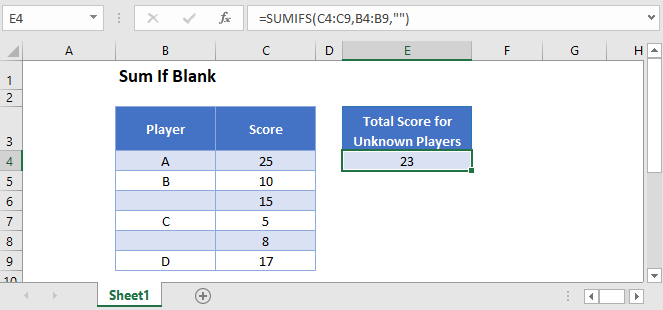## Sum if Blank

First, we will demonstrate how to sum rows with blank cells.

The SUMIFS Function sums data that meets certain criteria.

We can use the SUMIFS Function to sum all Scores for Players without names in the example below.

``=SUMIFS(C3:C8,B3:B8,"")``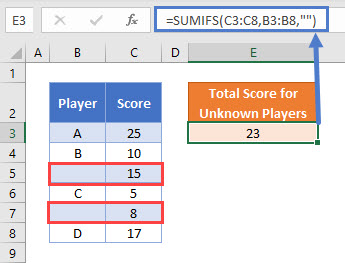We use double quotes (“”) to represent a blank cell in Excel. Our example ignores Players A, B, C, and D and sums the scores for unknown Players.

## Treating Spaces as Blank Cells – With Helper Column

You need to be careful when interacting with blank cells in Excel. Cells can appear blank to you, but Excel won’t treat them as blank. This can occur if the cell contains spaces, line-breaks, or other invisible characters. This is a common problem when importing data into Excel from other sources.

If we need to treat any cells that only contain spaces the same way as if they were blank, then the formula in the previous example will not work. Notice how the SUMIFS Formula does not consider cell B9 below (”   “) to be blank: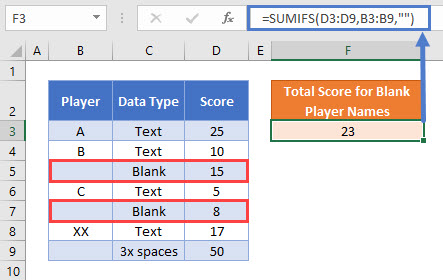To treat a cell containing only spaces as if it were a blank cell, we can add a helper column with the TRIM Function to remove the extra spaces from each cell’s value:

``=TRIM(B3)``We apply the SUMIFS Function to the helper column, and it now calculates the sum accurately.

``=SUMIFS(E3:E9,D3:D9,"")``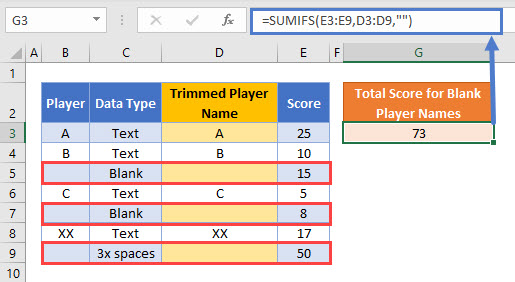The helper column is easy to create and easy to read, but you might wish to have a single formula to accomplish the task. This is covered in the next section.

## Treating Spaces as Blank Cells – Without Helper Column

If a helper column is not appropriate for your needs, then you can use the SUMPRODUCT Function in combination with the LEN and TRIM Functions to sum blank rows.

``=SUMPRODUCT(--(LEN(TRIM(B3:B9))=0),D3:D9)``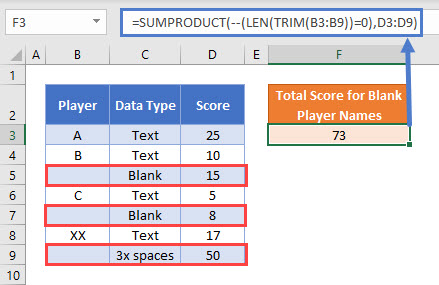In this example, we use the SUMPRODUCT Function to perform a complicated “sum if” calculation. Let’s walk through the formula.

This is our final formula:

``=SUMPRODUCT(--(LEN(TRIM(B3:B9))=0),D3:D9)``

First, the SUMPRODUCT Function lists the array of values from the two cell ranges:

``=SUMPRODUCT(--(LEN(TRIM({"A"; "B"; ""; "C"; ""; "XX"; "   "}))=0),{25; 10; 15; 5; 8; 17; 50})``

Then, the TRIM Function removes leading and trailing spaces from Player names:

``=SUMPRODUCT(--(LEN({"A"; "B"; ""; "C"; ""; "XX"; ""})=0),{25; 10; 15; 5; 8; 17; 50})``

The LEN Function calculates the lengths of the trimmed Player names:

``=SUMPRODUCT(--({1; 1; 0; 1; 0; 2; 0}=0),{25; 10; 15; 5; 8; 17; 50})``

With the logical test (=0), any trimmed Player names with 0 characters are changed to TRUE:

``=SUMPRODUCT(--({FALSE; FALSE; TRUE; FALSE; TRUE; FALSE; TRUE}),{25; 10; 15; 5; 8; 17; 50})``

Next the double dashes (–) convert the TRUE and FALSE values into 1s and 0s:

``=SUMPRODUCT({0; 0; 1; 0; 1; 0; 1},{25; 10; 15; 5; 8; 17; 50})``

The SUMPRODUCT Function then multiplies each pair of entries in the arrays to produce an array of Scores only for Player names that are blank or made only from spaces:

``=SUMPRODUCT({0; 0; 15; 0; 8; 0; 50})``

Finally, the numbers in the array are summed together:

``=73``

More details about using Boolean statements and the “–” command in a SUMPRODUCT Function can be found here.

## Sum If Blank in Google Sheets

These formulas work exactly the same in Google Sheets as in Excel.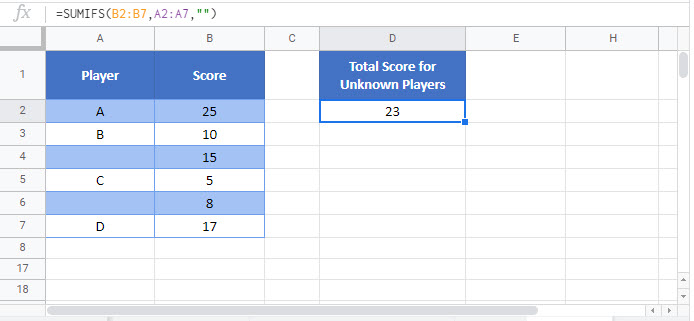### Excel Practice Worksheet

Practice Excel functions and formulas with our 100% free practice worksheets!